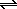Practice Problem 11

Use the following standard-state cell potentials to calculate the complex formation equilibrium constant for the Zn(NH3)42+ complex ion.

 Zn(NH3)42+ + 2 e-Zn + 4 NH3 Eored = -1.04 V Zn2+ + 2 e-Zn Eored = -0.7628 V

Answer

2.9 x 109